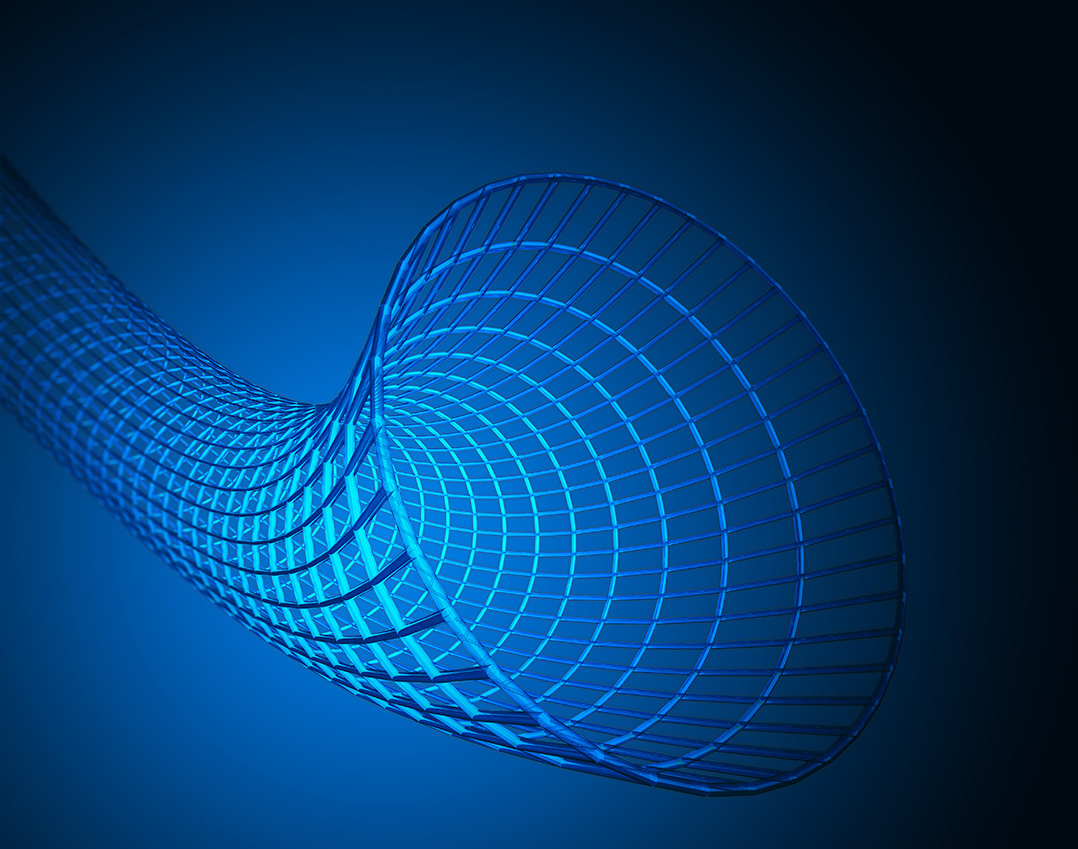# String TheoryString theory is an intricate web of physical and mathematical ideas encompassing a quantum theory of gravity, a conjectural unified description of particle interactions, and a multitude of connections to geometry and topology. It has a very rich underlying mathematical structure which makes contact with many areas of mathematics including representation theory, integrable systems, differential geometry, low dimensional topology and algebraic geometry.

The String Theory project addresses questions aiming to provide a better understanding of the underlying mathematical structures and questions using string theory as an inspiration for conjectures and results concerning the mathematics itself.

Research groups within the projects address questions that include:• Deepening understanding of the duality between quantum theories, geometry and gravity.
• Making aspects of duality  quantitively and structurally precise.
• Finding applications of duality to mathematics.

Year 6 Site Visit highlight project presentation video